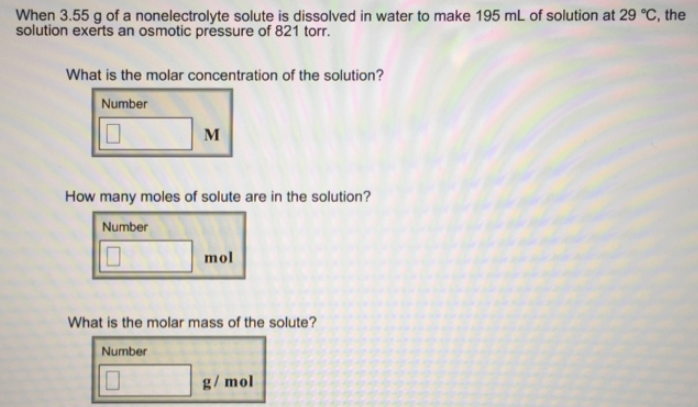# Problem: When 3.55 g of a nonelectrolyte solute is dissolved in water to make 195 mL of solution at 29 °C, the solution exerts an osmotic pressure of 821 torr. What is the molar concentration of the solution? How many moles of solute are in the solution? What is the molar mass of the solute?

###### FREE Expert Solution
86% (242 ratings)###### Problem Details

When 3.55 g of a nonelectrolyte solute is dissolved in water to make 195 mL of solution at 29 °C, the solution exerts an osmotic pressure of 821 torr.

What is the molar concentration of the solution?

How many moles of solute are in the solution?

What is the molar mass of the solute?